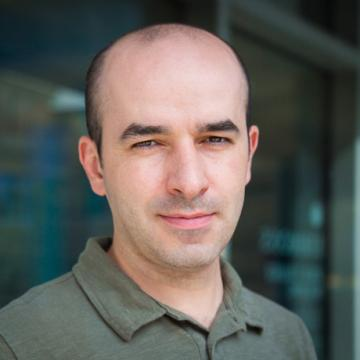# Viktor Grigoryan

Associate Professor
• Mathematics, Computing, and StatisticsS214

(617) 521-2491

## About Me

I am a Mathematical Analyst working in the area of Differential Equations, so the courses I teach span the spectrum of analysis courses from introductory calculus to upper division real analysis and differential equations. I am also interested in the applied aspects of analysis as well, and have taught topics courses in Mathematical Biology and Stochastic Processes.

Viktor Grigoryan's Curriculum Vitae

## What I Teach

• Math 101 - Introduction to Mathematics
• Math 120 - Calculus I
• Math 121 - Calculus II
• Math 123 - Single Variable Calculus
• Math 220 - Multivariable Calculus
• Math 225 - Differential Equations
• Math 320 - Introduction to Real Analysis I
• Math 321 - Introduction to Real Analysis II
• Math 390 - Mathematical Biology
• Math 391 - Random Processes

## Research/Creative Activities

The broad area of my research is analysis and applications of Partial Differential Equations (PDEs). On the analysis side I'm interested in understanding the existence and behavior of solutions to nonlinear wave and dispersive equations. These types of equations model propagation of energy and oscillatory wave behavior through different media and are useful in describing various phenomena from physics and geometry to chemistry and biology. On the applied side, I'm interested in computational modeling with differential equations with a special focus on biological systems and processes, such as wound healing, cardiac electrophysiology and populations dynamics.

I am also interested in mathematical pedagogy, especially incorporating active learning paradigms in teaching mathematics at institutions of higher education. To this end I have developed various resources for teaching real analysis, differential equations and multivariable calculus using the inquiry based learning methodology (IBL).